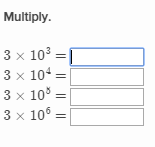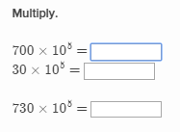The Multiplying and dividing whole numbers by powers of 10 exercise appears under the 5th grade (U.S.) Math Mission. This exercise strengthens the knowledge that multiplying and dividing by powers of ten moves the decimal point either left or right.

## Types of Problems

There are two types of problems in this exercise:

1. Multiply or divide the number by powers of ten: This problem asks several questions that involve multiplying or dividing a particular whole number by different powers of ten. The user is asked to fill in the correct answers.Multiply or divide the number by powers of ten
2. Multiply or divide the numbers by the power of ten: This problem provides a power of ten that is to be multiplied or divide against different whole numbers. The user is asked to provide all correct answers.Multiply or divide the numbers by the power of ten

## Strategies

Knowledge of the base ten number system and the result in this system when working with powers of ten is an advantage, but to complete the exercise all one needs is an ability to perform basic arithmetic operations.

1. In whole numbers the tacit decimal point is located at the end of the number.
2. Multiplying by a power of ten moves the decimal place to the right the same number of spaces as the exponent on the power of ten.
3. Dividing by a power of ten moves the decimal place to the left the same number of spaces as the exponent on the power of ten.

## Real-life Applications

1. Scientific notation uses powers of ten to represent large and small quantities in a more efficient way.
2. Powers of ten simplify comparison of numbers by order of magnitude.
3. Decimals are very common in shopping. Price tags, food labels, and receipts all contain decimals.
4. At gas pumps, decimals are used to show how much gas is pumped and much it costs per gallon.